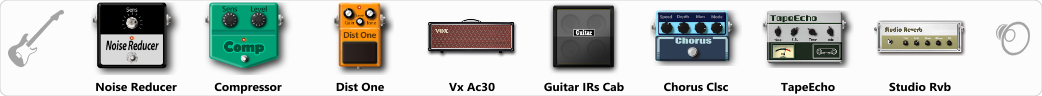Discussion in 'ToneLib-GFX presets' started by ktaxi, Apr 23, 2021.

Bridge pickup,ihope you like it!!!

Effects chain:Effect: "Noise Reducer" (Dynamics / Filter), active - "yes"
"Sens" = 65
"Mode" = Hard

Effect: "Compressor" (Dynamics / Filter), active - "yes"
"Sense" = 41
"Level" = 42

Effect: "Dist One" (Overdrive / Distortion), active - "yes"
"Drive" = 36
"Tone" = 62
"Level" = 71

Effect: "Vx Ac30" (Amp simulators), active - "yes"
"Gain" = 63
"Bass" = 43
"Middle" = 75
"Treble" = 54
"Presence" = 64
"Master" = 90
"Output" = 50
"Level (dB)" = 0

Effect: "Guitar IRs Cab" (Cabinets), active - "yes"
"Model" = Mesa Boogie Mk3 (1x12")
"Mic Position" = Middle
"Mic Distance" = Far
"Low Cut (Hz)" = 60
"Hi Cut (kHz)" = 20.0
"Mix" = 100
"Level (dB)" = 0

Effect: "Chorus Clsc" (Modulation / Sfx), active - "yes"
"Speed" = 1.7
"Depth" = 35
"Center" = 3.6
"Mode" = Stereo

Effect: "TapeEcho" (Delay), active - "yes"
"Time" = 395
"Feedback" = 20
"Tone" = 65
"LoDamp" = 19
"Mix" = 53

Effect: "Studio Rvb" (Reverb), active - "yes"
"Time" = 5.6
"PreDelay" = 32
"LoDamp" = 0
"HiDamp" = 12
"Mix" = 43

Note: You will need to download and install the ToneLib-GFX software to use the preset.